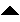Next: Conclusion Up: number8 Previous: The Z Transform

# Convolutions and Digital Filters

Having established the convolution theorem is very important since digital filters can be described in terms of convolutions. The Z transform version allows us to work with these filters by merely manipulating polynomials. In order to see that this is so, I have to reveal one neat fact about the Z transform. The Z transform that we have describe above can be written as,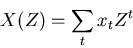(9)

If this is evaluated on the unit circle in the complex plane, that is we let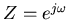, then we get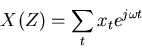(10)

which is the definition of the (discrete) Fourier transform! In other words the Fourier transform is just the Z transform evaluated on the unit circle. So one way to interpret (7), is that we can make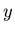be a series that has a spectrum that is the spectrum of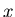modified by the spectrum of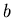. So for example if we designto have a zero at 60 Hz, thenwill be the signalwith 60 Hz removed, i.e. we have a notch filter.

In general we can write a digital filter in the form,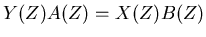(11)

or written out in the time domain,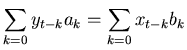(12)

Ifrepresents the measurement data and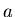andare the (somehow) known filter coefficients then the above equation can be reorganized in a way that is more obviously useful,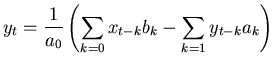(13)

This equation is a general formulation of a digital filter. If thecoefficients beyond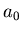are nonzero, it is called an Infinite Impluse Response (IIR) filter because an input seriesconsisting of all zeros except at one point will result in an output seriesthat will always contain some amount of the original input (for real computers with finite CELL sizes the output will actually eventually decay away because of truncation effects). An example IIR filter is what is known as the leaky integrator,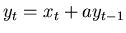For the special case where thecoefficients beyondare all zero, the filter is called a Finite Impluse Response (FIR) filter because an impulsive input will cause an output that will eventually settle down to a steady state. A simple example is the running average,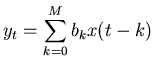Everything that one can know about a digital filter is contained in the ratio of elements the of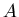and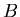,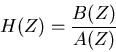(14)

which is known as the filter characteristic. Foremost is the power transfer function which describes what fraction of energy at a given frequency in the input appears in the output,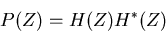(15)

where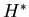is the complex conjugate (the sign of the imaginary part is inverted) of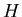.

Recall that in an earlier column, I pointed out the the power transfer function is only part of the story. One should also consider what the filter does to the phase of the input. In general a filters effect on the phase is frequency dependent and so there is a phase transfer function,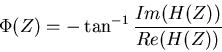(16)

The filter can also have an effect on packets of waves. These packets are what you get, for example, when you modulate a one frequency by another. The frequency of the modulation envelope itself becomes a filterable component with its own transfer function, the group transfer function,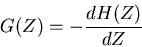(17)

All of these transfer functions are conventionally computed on the unit complex circle.

The characteristic function for the leaky integrator is,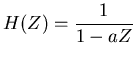Converting this to the time domain gives the impulse response of the filter,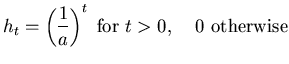For the running average filter, the function is,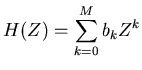and its impulse response is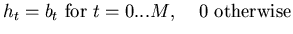Certain forms for the polynomialsandhave proven to be useful and are widely used. For example the bandpass Butterworth filter has the coefficients in the form,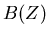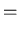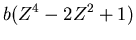(18)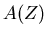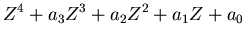The Butterworth filter chooses to optimize the flatness of the bandpass region of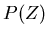and in doing so gives up simple forms for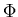and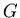.

Chebyshev filters are designed to give the sharpest possible transition between the bandpass and bandstop regions. The filter gets its name because the filter characteristic contains a special polynomial known as a Chebyshev polynomial,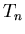.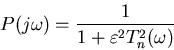(19)

This filter gains in the sharp transition by compromising on the flatness of the bandpass region.

Elliptical or Cauer filters get even sharper transitions than Chebyshev filters, but achieve this at the expense of ripples in both the pass and stop bands. The filter characteristic looks like the Chebyshev filter, except the Chebyshev polynomial gets replaced by a Chebyshev rational function.

Bessel filters are designed with the goal of achieving the flattest possible group delay. This results in a filter has no ringing when it receives a step or impulse input. The characteristic function for the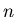th order Bessel filter is given by,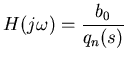(20)

where,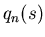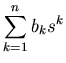(21)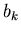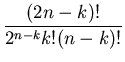Next: Conclusion Up: number8 Previous: The Z Transform
Skip Carter 2008-08-20